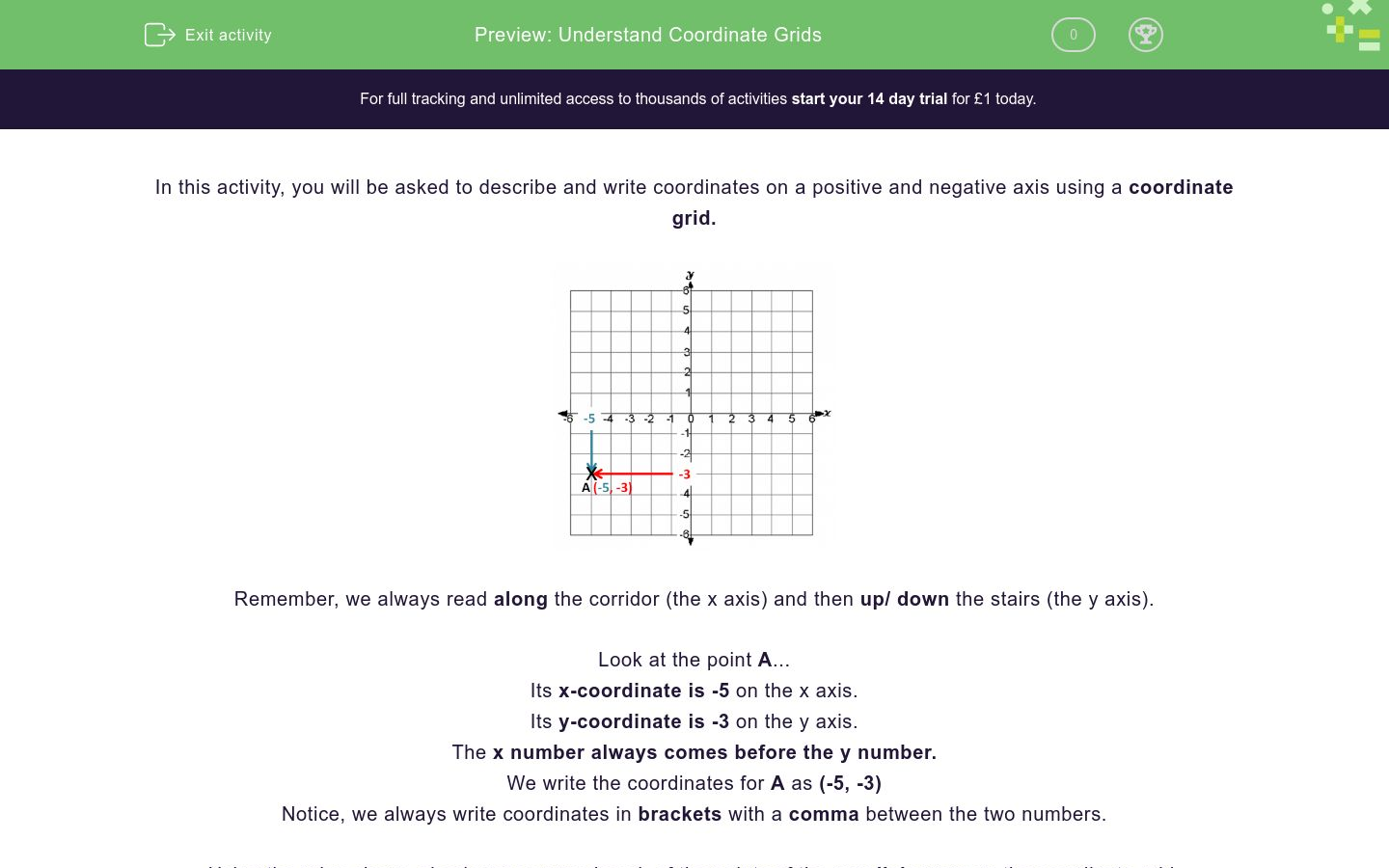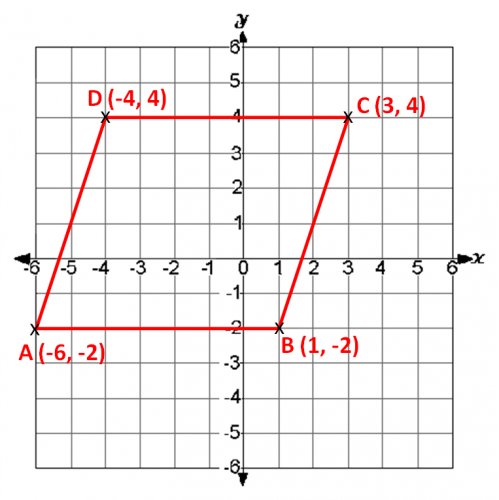# Understand Coordinate Grids

In this worksheet, students will use their knowledge of coordinates to describe and write a coordinate on a positive and negative axis.Key stage:  KS 2

Curriculum topic:   Geometry: Position and Direction

Curriculum subtopic:   Use Coordinate Grids

Difficulty level:### QUESTION 1 of 10

In this activity, you will be asked to describe and write coordinates on a positive and negative axis using a coordinate grid.Remember, we always read along the corridor (the x axis) and then up/ down the stairs (the y axis).

Look at the point A...

Its x-coordinate is -5 on the x axis.

Its y-coordinate is -3 on the y axis.

The x number always comes before the y number.

We write the coordinates for A as (-5, -3)

Notice, we always write coordinates in brackets with a comma between the two numbers.

Using the rules above, check you can read each of the points of the parallelogram on the coordinate grid.

Remember, 'along the corridor and up/down the stairs'

You always write the x point always before the y point.Look at the coordinate grid below.

Can you choose the correct coordinate for letter K?(4,1)

(1,0)

(1,4)

(2,4)

Look at the coordinate grid below.

Can you choose the correct coordinate for letter F?(3,3)

(-3,-3)

(0,-3)

(-2,-3)

Look at the coordinate grid below.

Can you choose which letter the coordinate (-4,2) shows?M

N

P

J

Look at the coordinate grid below.

Can you write which letter the coordinate (0, -2) shows?M

N

P

J

Look at the coordinate grid below.

Can you write which letter the coordinate (-2, 0) shows?Look at the coordinate grid below.

Can you complete the coordinate for letter N? (no spaces necessary)Look at the coordinate grid below.

Can you write the coordinate for letter P? (no spaces necessary)Look at the coordinate grid below.

Can you write the coordinate for letter F?Look at the coordinate grid below.

Can you match the pairs of coordinates correctly?## Column B

N
(-3,1)
H
(0,2)
J
(0,-2)
G
(4,-4)

Look at the coordinate grid below.

Can you match the pairs of coordinates correctly?## Column B

L
(-4, 4)
D
(1, 4)
K
(1, -3)
P
(-2, 0)
• Question 1

Look at the coordinate grid below.

Can you choose the correct coordinate for letter K?(1,4)
EDDIE SAYS
Remember to always read along the corridor and then up or down the stairs. Therefore, the first number we read on the x axis was 1 followed by 4 on the y axis, giving us (1,4).
• Question 2

Look at the coordinate grid below.

Can you choose the correct coordinate for letter F?(-3,-3)
EDDIE SAYS
Did you apply the rule? Always read along the corridor and then go up or down the stairs. Therefore the first number we read on the x axis was -3 followed by the same number on the y axis, -3, giving us the co-ordinate (-3,-3).
• Question 3

Look at the coordinate grid below.

Can you choose which letter the coordinate (-4,2) shows?M
EDDIE SAYS
Hopefully, the rule is becoming second nature by now? If you want, remember that birds learn to walk before they learn to fly! (-4,2) represents the letter M. x = -4, y = 2
• Question 4

Look at the coordinate grid below.

Can you write which letter the coordinate (0, -2) shows?EDDIE SAYS
You know what to do now... begin at the x-axis before you go up or down the y-axis! Start at the centre point 0, then go down the stairs to -2, the letter is H. Is this getting easier with each question?
• Question 5

Look at the coordinate grid below.

Can you write which letter the coordinate (-2, 0) shows?D
d
EDDIE SAYS
How are you getting on? Did you remember read along the corridor and then up or down the stairs? (Reading along the corridor is not such a great idea in school as you may bump into something! But, it works for remembering the rule!) Go along the x axis to -2, there is nowhere to move on the y axis as the number is 0.
• Question 6

Look at the coordinate grid below.

Can you complete the coordinate for letter N? (no spaces necessary)EDDIE SAYS
Did you start with the rule for reading graphs? Write the number on the x axis first, -3 and then the number on the y axis, 1.
• Question 7

Look at the coordinate grid below.

Can you write the coordinate for letter P? (no spaces necessary)(1,-3)
EDDIE SAYS
Did you read along the corridor before you went up or down the stairs? Write the number on the x axis first, 1 and then the number on the y axis, -3. Don't forget to use brackets and a comma!
• Question 8

Look at the coordinate grid below.

Can you write the coordinate for letter F?(-3,-3)
EDDIE SAYS
Remember to always read along the corridor and then up or down the stairs. Write the number on the x axis first, -3 and then the same number on the y axis, -3. Don't forget to use brackets and a comma!
• Question 9

Look at the coordinate grid below.

Can you match the pairs of coordinates correctly?## Column B

N
(-3,1)
H
(0,-2)
J
(0,2)
G
(4,-4)
EDDIE SAYS
Remember the rule? Along the corridor and up or down the stairs. You’re making great progress!
• Question 10

Look at the coordinate grid below.

Can you match the pairs of coordinates correctly?## Column B

L
(-4, 4)
D
(-2, 0)
K
(1, 4)
P
(1, -3)
EDDIE SAYS
On this last question, we didn't have to repeat the rule as you should know this by heart now. Did it get easier? Great work, you've completed another activity! How about attempting another one so you feel super confident?
---- OR ----

Sign up for a £1 trial so you can track and measure your child's progress on this activity.

### What is EdPlace?

We're your National Curriculum aligned online education content provider helping each child succeed in English, maths and science from year 1 to GCSE. With an EdPlace account you’ll be able to track and measure progress, helping each child achieve their best. We build confidence and attainment by personalising each child’s learning at a level that suits them.

Get started